The current in the given circuit is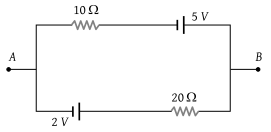(1) 0.1 A

(2) 0.2 A

(3) 0.3 A

(4) 0.4 A

Concept Questions :-

Kirchoff's voltage law
High Yielding Test Series + Question Bank - NEET 2020

Difficulty Level:

Consider four circuits shown in the figure below. In which circuit power dissipated is greatest (Neglect the internal resistance of the power supply)

(1)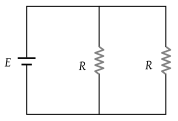(2)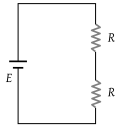(3)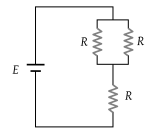(4)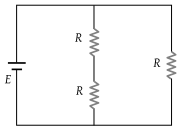Concept Questions :-

Heating effect of current
High Yielding Test Series + Question Bank - NEET 2020

Difficulty Level:

In the circuit, the reading of the ammeter is (assume internal resistance of the battery be zero) :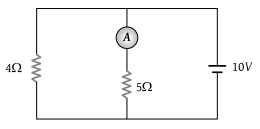(1) $\frac{40}{29}A$

(2) $\frac{10}{9}A$

(3) $\frac{5}{3}A$

(4) 2 A

Concept Questions :-

Kirchoff's voltage law
High Yielding Test Series + Question Bank - NEET 2020

Difficulty Level:

Eels are able to generate current with biological cells called electroplaques. The electroplaques in an eel are arranged in 100 rows, each row stretching horizontally along the body of the fish containing 5000 electroplaques. The arrangement is suggestively shown below. Each electroplaques has an emf of 0.15 V and internal resistance of 0.25 Ω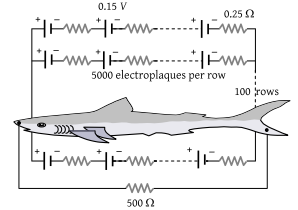The water surrounding the eel completes a circuit between the head and its tail. If the water surrounding it has a resistance of 500 Ω, the current an eel can produce in water is about

(1) 1.5 A

(2) 3.0 A

(3) 15 A

(4) 30 A

Concept Questions :-

Grouping of cells
High Yielding Test Series + Question Bank - NEET 2020

Difficulty Level:

A battery is charged at a potential of 15 V for 8 hours when the current flowing is 10 A. The battery on discharge supplies a current of 5 A for 15 hours. The mean terminal voltage during discharge is 14 V. The "Watt-hour" efficiency of the battery is :

(1) 82.5%

(2) 80 %

(3) 90%

(4) 87.5%

Concept Questions :-

Heating effect of current
High Yielding Test Series + Question Bank - NEET 2020

Difficulty Level:

In the given current distribution what is the value of I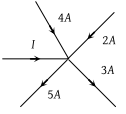(1) 3A

(2) 8 A

(3) 2A

(4) 5A

Concept Questions :-

Kirchoff's current law
High Yielding Test Series + Question Bank - NEET 2020

Difficulty Level:

A capacitor is connected to a cell of emf E having some internal resistance r. The potential difference across the

(1) Cell is < E

(2) Cell is E

(3) Capacitor is > E

(4) Capacitor is < E

Concept Questions :-

Grouping of cells
High Yielding Test Series + Question Bank - NEET 2020

Difficulty Level:

The maximum power drawn out of the cell from a source is given by (where r is internal resistance)

(1) ${E}^{2}/2r$

(2) ${E}^{2}/4r$

(3) ${E}^{2}/r$

(4) ${E}^{2}/3r$

Concept Questions :-

Heating effect of current
High Yielding Test Series + Question Bank - NEET 2020

Difficulty Level:

Find out the value of current through 2Ω resistance for the given circuit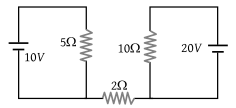(1) 5 A

(2) 2 A

(3) Zero

(4) 4 A

Concept Questions :-

Kirchoff's voltage law
High Yielding Test Series + Question Bank - NEET 2020

Difficulty Level:

Two sources of equal emf are connected to an external resistance R. The internal resistances of the two sources are R1 and ${R}_{2}\text{\hspace{0.17em}}\left({R}_{2}>{R}_{1}\right)$. If the potential difference across the source having internal resistance R2 is zero, then

(1) $R={R}_{1}{R}_{2}/\left({R}_{1}+{R}_{2}\right)$

(2) $R={R}_{1}{R}_{2}/\left({R}_{2}-{R}_{1}\right)$

(3) $R={R}_{2}×\left({R}_{1}+{R}_{2}\right)/\left({R}_{2}-{R}_{1}\right)$

(4) $R={R}_{2}-{R}_{1}$

Concept Questions :-

Grouping of cells